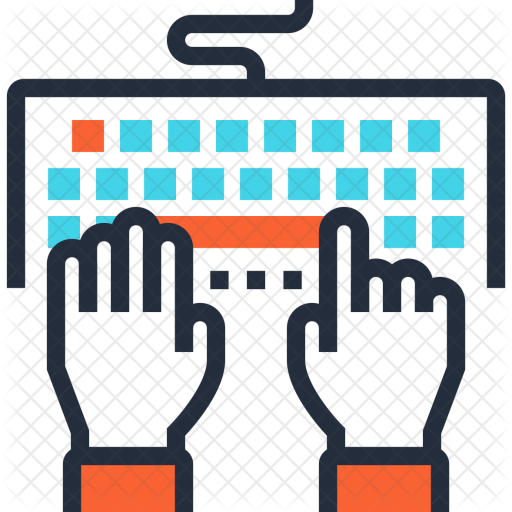# The Tidyverse and dplyr

In this lesson, you’ll learn how to wrangle data using the dplyr package in the tidyverse

When you are finished, you should be able to…

• Understand what the tidyverse is

• Use the pipe operator (%>%)

• Use the five main dplyr verbs:

• filter()

• arrange()

• select()

• mutate()

• summarize()

• Use group_by() to perform groupwise operationsTime Estimates:Videos: 40 minReadings: 0-60 minActivities: 20 minCheck-ins: 1

## What is the Tidyverse?Required Video: Intro to the TidyverseOptional Video: The beginning of the word ‘tidyverse’

## Wrangling data with dplyrRequired Reading: TibblesRequired Video: dplyrSee the slides.Recommended Reading: Data WranglingRecommended Reading: Data TransformationRecommended Tutorial: Practice with DplyrCheck-In 1: dplyr

Question 1: Suppose we would like to study how the ratio of penguin body mass to flipper size differs across the species. Rearrange the following steps in the pipeline into an order that accomplishes this goal.

# a
arrange(avg_mass_flipper_ratioo)

# b
group_by(species)

# c
penguins

# d
summarize(
avg_mass_flipper_ratioo = median(mass_flipper_ratio)
)

# e
mutate(
mass_flipper_ratio = body_mass_g/flipper_length_mm
)

Question 2:

Consider the base R code below.

mean(penguins[penguins\$species == "Adelie", "body_mass_g"])

For each of the following dplyr pipelines, indicate if it

1. Returns the exact same thing as the Base R code;
2. Returns the correct information, but the wrong object type;
3. Returns incorrect information; or
4. Returns an error
# a
penguins %>%
filter("body_mass_g") %>%
mean()

# b
penguins %>%
select(body_mass_g) %>%
summarize(mean(body_mass_g))

# c
penguins %>%
pull(body_mass_g) %>%
mean()

# d
penguins %>%
select(body_mass_g) %>%
mean()

# e
penguins %>%
summarize(mean(body_mass_g))
## Walkthrough of cereals activityOptional Video: Live coding of cereals dataset